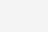# WebRTC ANR流程解析(WebRTC的单通道降噪方法)

## I. 维纳滤波器(Wiener Filter)其中

WebRTC的NS大致流程为通过计算先验和后验SNR来估计每个频段噪声存在的概率，然后对噪声估计进行更新，得到噪声谱后更新维纳滤波器的系数与输入信号结合输出干净的信号。下面详细介绍每个步骤。

## II. 初始化 Initialize

WebRTC NS初始化的东西比较多，按函数顺序来。

``int WebRtcNs_InitCore(NoiseSuppressionC* self, uint32_t fs)``

``static void set_feature_extraction_parameters(NoiseSuppressionC* self)``

``int WebRtcNs_set_policy_core(NoiseSuppressionC* self, int mode)``

## III. Analyze

``void WebRtcNs_AnalyzeCore(NoiseSuppressionC* self, const float* speechFrame) ``

``````
static void UpdateBuffer(const int16_t *frame,
size_t frame_length,
size_t buffer_length,
float *buffer) ``````

``````static float WindowingEnergy(const float *window,
const float *data,
size_t length,
float *data_windowed)``````

``````static void FFT(NoiseSuppressionC *self,
float *time_data,
size_t time_data_length,
size_t magnitude_length,
float *real,
float *imag,
float *magn, float *lmagn, int prev_calc, float *signalEnergy, float *sumMagn)``````

``````static void NoiseEstimation(NoiseSuppressionC *self,
float *lmagn,
float *noise)``````

``````
static void ComputeSnr(const NoiseSuppressionC *self,
const float *magn,
const float *noise,
float *snrLocPrior, float *logSnrLocPrior,
float *snrLocPost) {
size_t i;

for (i = 0; i < self->magnLen; i++) {
// Previous post SNR.
// Previous estimate: based on previous frame with gain filter.
float previousEstimateStsa = (self->magnPrevAnalyze[i] * self->smooth[i]) / (self->noisePrev[i] + epsilon);
// Post SNR.
snrLocPost[i] = 0.f;
if (magn[i] > noise[i]) {
snrLocPost[i] = (magn[i] - noise[i]) / (noise[i] + epsilon);
}
// DD estimate is sum of two terms: current estimate and previous estimate.
// Directed decision update of snrPrior.
snrLocPrior[i] = 2.f * (
DD_PR_SNR * previousEstimateStsa + (1.f - DD_PR_SNR) * snrLocPost[i]);
logSnrLocPrior[i] = log1pf(snrLocPrior[i]);
}  // End of loop over frequencies.
}``````

``````static void FeatureUpdate(NoiseSuppressionC *self,
const float *magn, const float *lmagn,
int updateParsFlag)``````

``````static void SpeechNoiseProb(NoiseSuppressionC *self,
float *probSpeechFinal,
const float *snrLocPrior, const float *logSnrLocPrior,
const float *snrLocPost)``````

``````static void UpdateNoiseEstimate(NoiseSuppressionC *self,
const float *magn,
float *noise)``````

## IV. Process

``````static void UpdateBuffer(const int16_t *frame,
size_t frame_length,
size_t buffer_length,
float *buffer) ``````
``````
static float WindowingEnergy(const float *window,
const float *data,
size_t length,
float *data_windowed)``````

``````static void ComputeDdBasedWienerFilter(const NoiseSuppressionC *self,
const float *magn,
float *theFilter) {
size_t i;
float snrPrior, previousEstimateStsa, currentEstimateStsa;

for (i = 0; i < self->magnLen; i++) {
// Previous estimate: based on previous frame with gain filter.
previousEstimateStsa = self->magnPrevProcess[i] * self->smooth[i] / (self->noisePrev[i] + epsilon);
// Post and prior SNR.
currentEstimateStsa = 0.f;
if (magn[i] > self->noise[i]) {
currentEstimateStsa = (magn[i] - self->noise[i]) / (self->noise[i] + epsilon);
}
// DD estimate is sum of two terms: current estimate and previous estimate.
// Directed decision update of |snrPrior|.
snrPrior = DD_PR_SNR * previousEstimateStsa +
(1.f - DD_PR_SNR) * currentEstimateStsa;
// Gain filter.
theFilter[i] = snrPrior / (self->overdrive + snrPrior);
}  // End of loop over frequencies.
}``````

``````self->smooth[i] = theFilter[i];
real[i] *= self->smooth[i];
imag[i] *= self->smooth[i];``````

``````static void IFFT(NoiseSuppressionC *self,
const float *real,
const float *imag,
size_t magnitude_length,
size_t time_data_length,
float *time_data) ``````

## V. Conclusion

. 实时语音处理实践指南

. Speech Enhancement Theory and Practice

. https://blog.csdn.net/golfbears/article/details/91882680

(0)